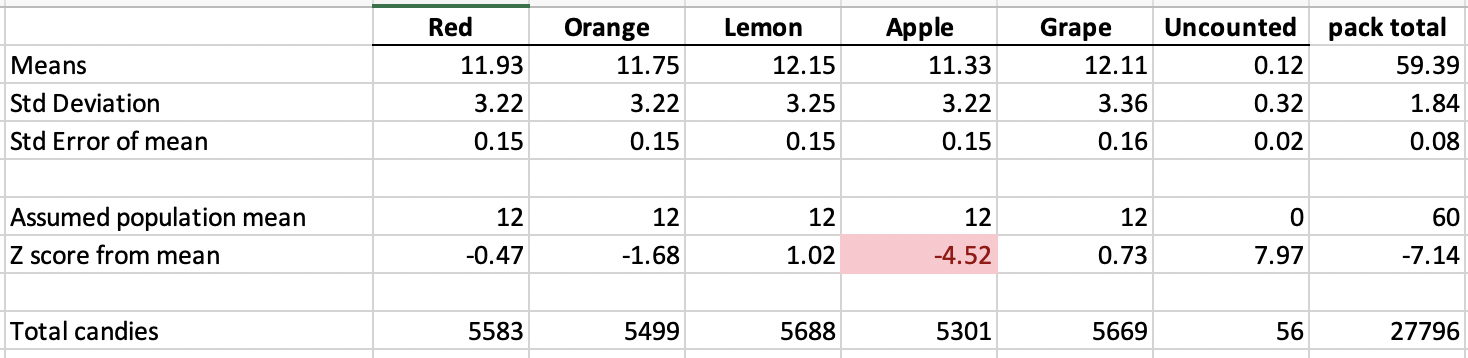# Never enough… red skittles

Red skittles are scarce. At least that’s always been my impression every time I open a mini pack we get as halloween candy. Too many yellows, too many greens. Never enough reds.  Possiblywrong recently published some data on 468 packs of skittles looking for duplicate packs. I wanted to use his research data to answer a different question: Are red skittles scarce compared to the other colors?

Using his data and the statistical analysis tools given to me by my MBA professor (Robert Dauffenbach) we can answer this question.  First the raw data- in 468 packs of skittles there were 5583 reds, 5499 oranges, 5688 yellows, 5301 greens, and 5669 purples.  Averaged out it is real close to 12 skittles of each color in a pack.Close to uniform, but not exactly uniform.  Let’s analyze this further:

From his raw data the standard deviations of number of candies by color is about 3.2.  Here is the compiled data:So, true population averages appear to be 12 candies of each color with a standard deviation of 3.2 per color (in one bag). Now the central limit theorem will help us here- even though the underlying distribution is uniform, a distribution of sample averages will be normal, assuming n is sufficiently large, and n=468 definitely satisfies this requirement (n>=30 is probably all we need to get from uniform parent to normal sampled means).   Now if we make the assumption that the population means and standard deviations are 12 and 3.25 respectively, we can answer the question if the difference in Yellows (12.15) to Reds (11.93) is statistically significant.  The standard error of the mean is the standard deviation over the square root of the number of samples = 3.25/sqrt(468) = 0.15.   That means if we have have 12.15 yellows, that’s one standard error of the mean from 12, a result we should find 68% of the time.  In other words not statistically significant.   (Busted — yellows are not more common).  Reds with a z-score of -0.47 are also within 1 standard deviation of the standard error of the mean meaning reds are plentiful — they are not held back, regardless of what I think.

However, the data does point to one outlier — greens. At an average of 11.33 that is -4.52 standard deviations below what’s expected.  The probability associated with a z-score of -4.5 is about 1/100,000 — meaning if it was a daily possibility you expect to find it 1 day in 275 years.  That is statistically significant and the null hypothesis that greens are filled at 12 per pack is rejected.  The alternative hypothesis is accepted, and that implication is that skittles intentionally under-fills green in order to keep packs at 59 per pack and not at 60.

Who would have thunk it? Greens!!